# Get Started

Starting with rasterpic is very easy! You just need a image (png, jpeg/jpg or tif/tiff) and a spatial object (from the sf or the terra) package to start using it.

## Basic usage

We use here as an example the shape of Austria:

library(sf)
library(terra)
library(rasterpic)

package = "rasterpic"
),
quiet = TRUE
)
img <- system.file("img/vertical.png",
package = "rasterpic"
)

# Create!

default <- rasterpic_img(x, img)

plotRGB(default)
plot(st_geometry(x), add = TRUE, col = "grey90")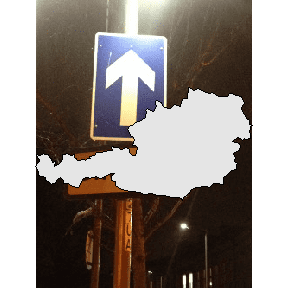## Options

The function provides several options for expanding, alignment and cropping.

### Expand

With this option the image is zoomed out of the spatial object:

expand <- rasterpic_img(x, img, expand = 1)

plotRGB(expand)
plot(st_geometry(x), add = TRUE, col = "grey90")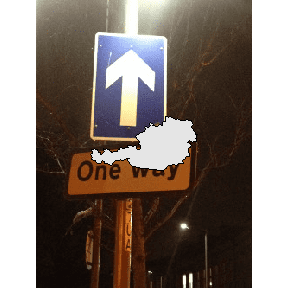### Alignment

Decide where to align the image:

bottom <- rasterpic_img(x, img, valign = 0)

plotRGB(bottom)
plot(st_geometry(x), add = TRUE, col = "grey90")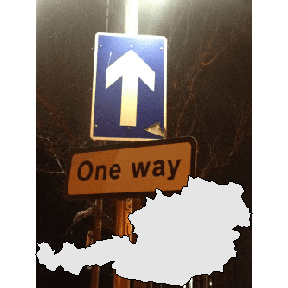Create impressive maps!:

mask <- rasterpic_img(x, img, crop = TRUE, mask = TRUE)

plotRGB(mask)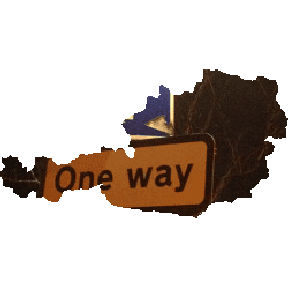crop = TRUE,
inverse = TRUE
)

plotRGB(maskinverse)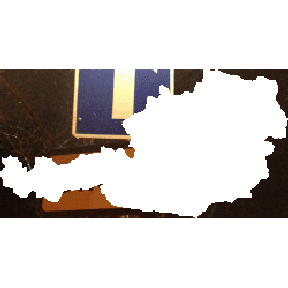• Spatial object of the sf package: sf, sfc, sfgor bbox.

• Spatial objects of the terra package: SpatRaster, SpatVector, SpatExtent.

• A vector of coordinates with the form c(xmin, ymin, xmax, yman)

• png files.
• jpg/jpeg files.
• tif/tiff files.